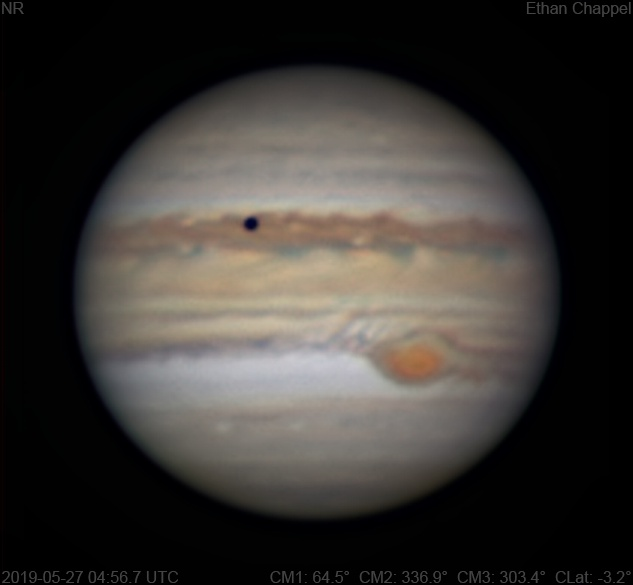# Jupiter 2019-05-27 04:56 UTC

CM1: 64.50°

CM2: 336.90°

CM3: 303.40°

CLat: -3.20°

Description

I wasn't expecting to image within the next week. However, I found Jupiter shining through a very thin cloud layer, so I hastily set up the C8 in a race against the clouds that were predicted to arrive at any moment. This was the first light of my new ZWO RGB filters, which I find to be much better than the Orion filters I was previously using with the this telescope.

Notes:

• Io and its shadow is transiting the North Equatorial Belt.
• White Oval Z is directly north of the Great Red Spot.
• The Equatorial Zone is still orange. The southern edge appears to be finally losing its white color.
• The South Equatorial Belt appears to be split by a white zone. The southern edge of the belt appears more gray than brown.
• The Great Red Spot has a large flake on its western edge.
• Another cloud flaked off the GRS a few days earlier is to the east of the storm.

Equipment

ZWO ASI174MM

Celestron X-Cel 3x

Celestron EdgeHD 8

Logs
```FireCapture v2.6  Settings
------------------------------------
Observer=Ethan Chappel
Camera=ZWO ASI174MM
Filter=B
Profile=Jupiter
Diameter=45.56"
Magnitude=-2.58
CMI=71.3° CMII=343.7° CMIII=310.1°  (during mid of capture)
FocalLength=7900mm (F/28)
Resolution=0.15"
Filename=2019-05-27-0508_1-EC-B-Jup.ser
Date=2019_05_27
Start=05_07_12.583
Mid=05_08_09.763
End=05_09_06.943
Start(UT)=05_07_12.583
Mid(UT)=05_08_09.763
End(UT)=05_09_06.943
Duration=114.360s
Date_format=yyyy_MM_dd
Time_format=HH_mm_ss
LT=UT -6h
Frames captured=2263
File type=SER
Binning=no
Bit depth=8bit
Debayer=no
ROI=464x460
ROI(Offset)=0x0
FPS (avg.)=19
Shutter=35.69ms
Gain=374 (93%)
FPS=100 (off)
HighSpeed=off
USBTraffic=80 (off)
Gamma=50 (off)
AutoGain=off
AutoExposure=off
SoftwareGain=10 (off)
AutoHisto=75 (off)
Brightness=1 (off)
Histogramm(min)=0
Histogramm(max)=128
Histogramm=50%
Noise(avg.deviation)=2.01
AutoAlign=false
PreFilter=none
Limit=120 Seconds
Sensor temperature=36.1°C
FireCapture v2.6  Settings
------------------------------------
Observer=Ethan Chappel
Camera=ZWO ASI174MM
Filter=G
Profile=Jupiter
Diameter=45.56"
Magnitude=-2.58
CMI=69.5° CMII=341.9° CMIII=308.4°  (during mid of capture)
FocalLength=8000mm (F/28)
Resolution=0.15"
Filename=2019-05-27-0505_2-EC-G-Jup.ser
Date=2019_05_27
Start=05_04_13.318
Mid=05_05_13.324
End=05_06_13.330
Start(UT)=05_04_13.318
Mid(UT)=05_05_13.324
End(UT)=05_06_13.330
Duration=120.012s
Date_format=yyyy_MM_dd
Time_format=HH_mm_ss
LT=UT -6h
Frames captured=7743
File type=SER
Binning=no
Bit depth=8bit
Debayer=no
ROI=464x460
ROI(Offset)=0x0
FPS (avg.)=64
Shutter=15.45ms
Gain=400 (100%)
FPS=100 (off)
HighSpeed=off
USBTraffic=80 (off)
Gamma=50 (off)
AutoGain=off
AutoExposure=off
SoftwareGain=10 (off)
AutoHisto=75 (off)
Brightness=1 (off)
Histogramm(min)=0
Histogramm(max)=147
Histogramm=57%
Noise(avg.deviation)=2.69
AutoAlign=false
PreFilter=none
Limit=120 Seconds
Sensor temperature=36.2°C
FireCapture v2.6  Settings
------------------------------------
Observer=Ethan Chappel
Camera=ZWO ASI174MM
Filter=R
Profile=Jupiter
Diameter=45.56"
Magnitude=-2.58
CMI=67.7° CMII=340.1° CMIII=306.6°  (during mid of capture)
FocalLength=7900mm (F/28)
Resolution=0.15"
Filename=2019-05-27-0502_2-EC-R-Jup.ser
Date=2019_05_27
Start=05_01_16.679
Mid=05_02_16.680
End=05_03_16.682
Start(UT)=05_01_16.679
Mid(UT)=05_02_16.680
End(UT)=05_03_16.682
Duration=120.003s
Date_format=yyyy_MM_dd
Time_format=HH_mm_ss
LT=UT -6h
Frames captured=3994
File type=SER
Binning=no
Bit depth=8bit
Debayer=no
ROI=464x460
ROI(Offset)=0x0
FPS (avg.)=33
Shutter=30.00ms
Gain=374 (93%)
FPS=100 (off)
HighSpeed=off
USBTraffic=80 (off)
Gamma=50 (off)
AutoGain=off
AutoExposure=off
SoftwareGain=10 (off)
AutoHisto=75 (off)
Brightness=1 (off)
Histogramm(min)=0
Histogramm(max)=125
Histogramm=49%
Noise(avg.deviation)=1.98
AutoAlign=false
PreFilter=none
Limit=120 Seconds
Sensor temperature=33.6°C
FireCapture v2.6  Settings
------------------------------------
Observer=Ethan Chappel
Camera=ZWO ASI174MM
Filter=B
Profile=Jupiter
Diameter=45.56"
Magnitude=-2.58
CMI=60.9° CMII=333.4° CMIII=299.8°  (during mid of capture)
FocalLength=7400mm (F/26)
Resolution=0.16"
Filename=2019-05-27-0451_0-EC-B-Jup.ser
Date=2019_05_27
Start=04_50_12.609
Mid=04_51_04.733
End=04_51_56.858
Start(UT)=04_50_12.609
Mid(UT)=04_51_04.733
End(UT)=04_51_56.858
Duration=104.249s
Date_format=yyyy_MM_dd
Time_format=HH_mm_ss
LT=UT -6h
Frames captured=1556
File type=SER
Binning=no
Bit depth=8bit
Debayer=no
ROI=464x460
ROI(Offset)=0x0
FPS (avg.)=14
Shutter=38.58ms
Gain=400 (100%)
FPS=100 (off)
HighSpeed=off
USBTraffic=80 (off)
Gamma=50 (off)
AutoGain=off
AutoExposure=off
SoftwareGain=10 (off)
AutoHisto=75 (off)
Brightness=1 (off)
Histogramm(min)=0
Histogramm(max)=168
Histogramm=65%
Noise(avg.deviation)=2.74
AutoAlign=false
PreFilter=none
Limit=120 Seconds
Sensor temperature=36.2°C
FireCapture v2.6  Settings
------------------------------------
Observer=Ethan Chappel
Camera=ZWO ASI174MM
Filter=G
Profile=Jupiter
Diameter=45.56"
Magnitude=-2.58
CMI=59.2° CMII=331.6° CMIII=298.1°  (during mid of capture)
FocalLength=7850mm (F/28)
Resolution=0.15"
Filename=2019-05-27-0448_2-EC-G-Jup.ser
Date=2019_05_27
Start=04_47_12.987
Mid=04_48_12.990
End=04_49_12.994
Start(UT)=04_47_12.987
Mid(UT)=04_48_12.990
End(UT)=04_49_12.994
Duration=120.007s
Date_format=yyyy_MM_dd
Time_format=HH_mm_ss
LT=UT -6h
Frames captured=5454
File type=SER
Binning=no
Bit depth=8bit
Debayer=no
ROI=464x460
ROI(Offset)=0x0
FPS (avg.)=45
Shutter=21.96ms
Gain=400 (100%)
FPS=100 (off)
HighSpeed=off
USBTraffic=80 (off)
Gamma=50 (off)
AutoGain=off
AutoExposure=off
SoftwareGain=10 (off)
AutoHisto=75 (off)
Brightness=1 (off)
Histogramm(min)=0
Histogramm(max)=125
Histogramm=49%
Noise(avg.deviation)=2.68
AutoAlign=false
PreFilter=none
Limit=120 Seconds
Sensor temperature=35.5°C
FireCapture v2.6  Settings
------------------------------------
Observer=Ethan Chappel
Camera=ZWO ASI174MM
Filter=R
Profile=Jupiter
Diameter=45.56"
Magnitude=-2.58
CMI=57.4° CMII=329.9° CMIII=296.3°  (during mid of capture)
FocalLength=7700mm (F/27)
Resolution=0.16"
Filename=2019-05-27-0445_2-EC-R-Jup.ser
Date=2019_05_27
Start=04_44_15.883
Mid=04_45_15.886
End=04_46_15.889
Start(UT)=04_44_15.883
Mid(UT)=04_45_15.886
End(UT)=04_46_15.889
Duration=120.006s
Date_format=yyyy_MM_dd
Time_format=HH_mm_ss
LT=UT -6h
Frames captured=1527
File type=SER
Binning=no
Bit depth=8bit
Debayer=no
ROI=464x460
ROI(Offset)=0x0
FPS (avg.)=12
Shutter=47.97ms
Gain=339 (84%)
FPS=100 (off)
HighSpeed=off
USBTraffic=80 (off)
Gamma=50 (off)
AutoGain=off
AutoExposure=off
SoftwareGain=10 (off)
AutoHisto=75 (off)
Brightness=1 (off)
Histogramm(min)=0
Histogramm(max)=133
Histogramm=52%
Noise(avg.deviation)=1.35
AutoAlign=false
PreFilter=none
Limit=120 Seconds
Sensor temperature=33.6°C
```
Measurements
Name Longitude 1 Longitude 2 Longitude 3 Latitude Measurer
GRS P. End 31.1° 303.6° 270° -21.9° EGC
GRS N. Edge 38.1° 310.5° 277° -17.6° EGC
GRS S. Edge 37.5° 310° 276.4° -26.5° EGC
GRS F. End 43.9° 316.3° 282.8° -22.6° EGC
GRS Center 38.1° 310.5° 277° -22.2° EGC